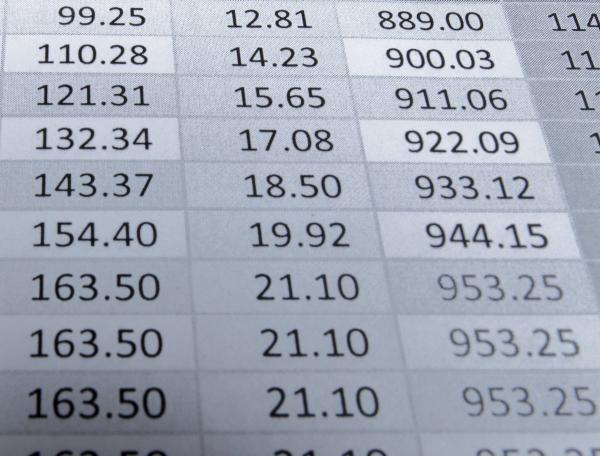# Decimal match-up

8 - 10 yearsDeveloped in partnership with Education Services Australia

The Australian Curriculum sets the goal for what all students should learn as they progress through their school life. Skills in the Year 3-4 curriculum include:

• visualising, describing and ordering tenths and hundredths
• visualising, describing and ordering 1-place and 2-place decimals
• knowing how to read decimals
• recognising that tenths written as fractions and as 1-place decimals have the same value
• recognising that hundredths written as fractions and as 2-place decimals have the same value
• solving problems using equivalent fractions for tenths, hundredths, 1-place and 2-place decimals.

It’s easy to help your child practise these skills as part of everyday life – just use these simple ideas.

## Decipher decimals

To master decimals, your child first needs to learn how they are read differently in different situations:

• 1.52 is read as one point five two or one and 52-hundredths
• \$1.52 is read as one dollar and fifty-two cents or one dollar fifty-two
• on a digital clock, 1:52 is ready as one fifty-two (and there are only 8 minutes until 2:00, not 48 minutes).

• read and measure decimal measurements
We need to cut 1.6m of ribbon, can you help me measure it?
How much are mangoes this week?
• read the time on digital clocks
• convert decimals into fractions
Our ceiling is 2.4 metres high, what's that as a fraction? Two and four tenths of a metre, that's right!
What's 7.99 as a fraction? Seven and ninety-nine hundredths!
• convert tenths and hundredths into decimals
The suitcase weighs nine and two tenths of a kilo - that's 9.2 kilos.
This measures one and seventy-one hundredths. What's that as a decimal? (Answer: 1.71)

## Go online

For online reinforcement, Decimaster: match-up will give your child practice at:

• representing tenths as decimals, fractions and on a grid
• connecting the different ways to represent tenths.

Wishball: Hundredths will give your child practice at:

• understanding place value (tens, ones, tenths and hundredths)
• doing simple addition and subtraction with decimals.

[3-4Learning]

Toolkits
Tags